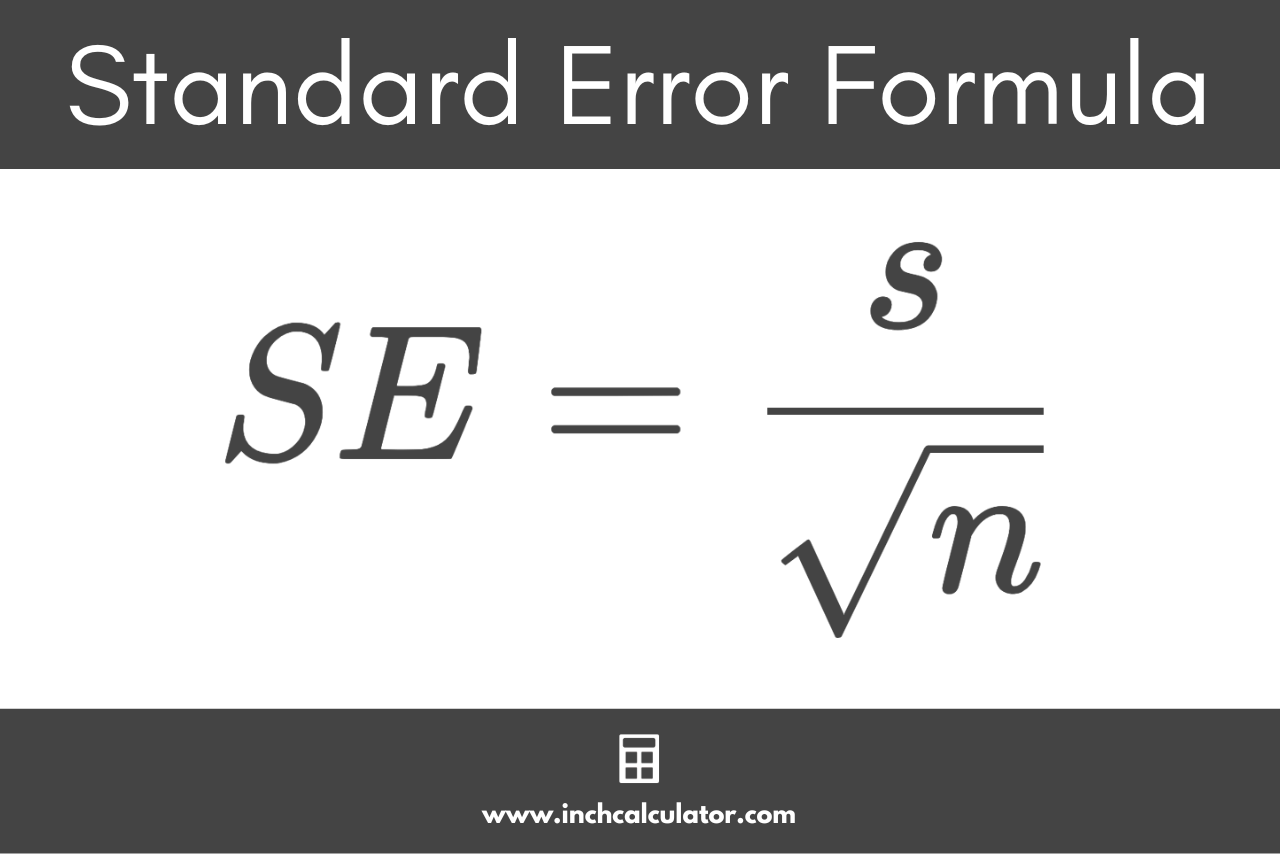# Standard Error Calculator

Find the standard error for your data set by entering the numbers in the calculator below.

Separate numbers using a comma (,)

## Results:

### Steps to Solve

#### Step One: Find the Sample Size

The sample size is the count of the values in the sample.

#### Step Two: Find the Standard Deviation

Use the standard deviation formula to solve for s.

s = \sqrt{\frac{\sum \left ( x_{i}-\bar{x} \right )^{2}}{n-1}}

#### Step Three: Find the Standard Error

Apply the standard error formula to solve for SE.

SE = \frac{s}{\sqrt{n}}
Learn how we calculated this below

## How to Find the Standard Error

Standard error is a measure of the accuracy of a measurement from a sample. More specifically, it’s a measure of how close the sample mean is to the population mean.

Standard error is denoted SE, and sometimes SEM since it’s also referred to as the standard error of the mean. SE is often used to calculate confidence intervals.

While both standard deviation and standard error are measures of variability, standard deviation is a measure of the dispersion of individual data values, and SE is a measure of the dispersion of the means.

### Standard Error Formula

The formula to calculate the standard error is:

SE = \frac{s}{\sqrt{n}}

Where
SE = standard error
s = sample standard deviation
n = sample size

Thus, the standard error is equal to the standard deviation divided by the square root of the sample size.Using the standard error formula, you can solve for SE in a few simple steps.

### Step One: Calculate the Sample Size

The sample size is simply the count of the values. You can find it by counting the number of elements in the data set.

### Step Two: Find the Standard Deviation

You can find the standard deviation for the sample using the following formula:

s = \sqrt{\frac{\sum \left ( x_{i}-\bar{x} \right )^{2}}{n-1}}

To solve for s, you’ll need to find the mean, sum of squares, and variance.

### Step Three: Find the Standard Error

Then, using the standard deviation, you can find the standard error using the formula above.

For example, let’s find the standard error given a sample size of 20 and a standard deviation of 1.73

SE = \frac{1.73}{\sqrt{20}}
SE = \frac{1.73}{4.47}
SE = 0.387

In this example, the standard error SE is equal to 0.387.

## References

1. Tuovila, A., Standard Error of the Mean vs. Standard Deviation: What's the Difference?, Investopedia, https://www.investopedia.com/ask/answers/042415/what-difference-between-standard-error-means-and-standard-deviation.asp
2. Altman, D., Standard deviations and standard errors, BMJ, Oct 15, 2005, 331(7521), 903. https://www.ncbi.nlm.nih.gov/pmc/articles/PMC1255808/